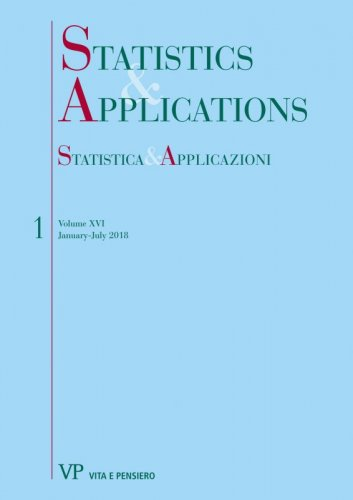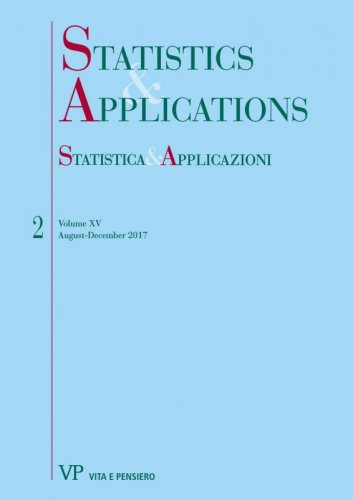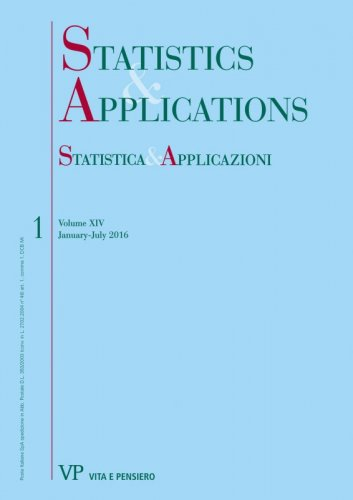Your browser does not support JavaScript!

Igor Valli

Author's books

Joint decomposition by subpopulations and sources of the point and synthetic Gini indexes newdigitalformat:Article| STATISTICA & APPLICAZIONI - 2018 - 1
Year: 2018
The decompositions proposed in this paper are applied to the net disposable income of the 8156 italian households supplied by Bank of Italy (2016) where the households are partitioned in four subpopulations according to the number of family members and the total income is the sum of four sources...
Joint decomposition by subpopulations and sources of the point and synthetic Bonferroni inequality measures digitalformat:Article| STATISTICA & APPLICAZIONI - 2017 - 2
Year: 2017
The total income Y is the sum of c sources Xj : Y = X1 + . . . + Xc: The N units of the population are partitioned in k different subpopulations. In the frequency distribution framework the Bonferroni (1930) point inequality index is given by Vh(Y) = [M(Y) - ̅Mh.(Y)]/M(Y), M(Y) and ̅Mh.(Y)are the mean and the lower mean of Y...
On the decomposition by subpopulations of the point and synthetic Bonferroni inequality measures digitalformat:Article| STATISTICA & APPLICAZIONI - 2016 - 1
Year: 2016
This paper, by using the ‘‘two-step’’ approach proposed in Radaelli (2008, 2010) and in Zenga (2016) for the decomposition of the Zenga (2007) index, obtains the decomposition of the Bonferroni (1930) inequality measure. In the first step the Bonferroni point measure Vh(Y) is decomposed in a weighted mean of k x k relative differences between the mean Mg(Y) of subpopulation g and the lower mean Mhl(Y) of the subpopulation l...